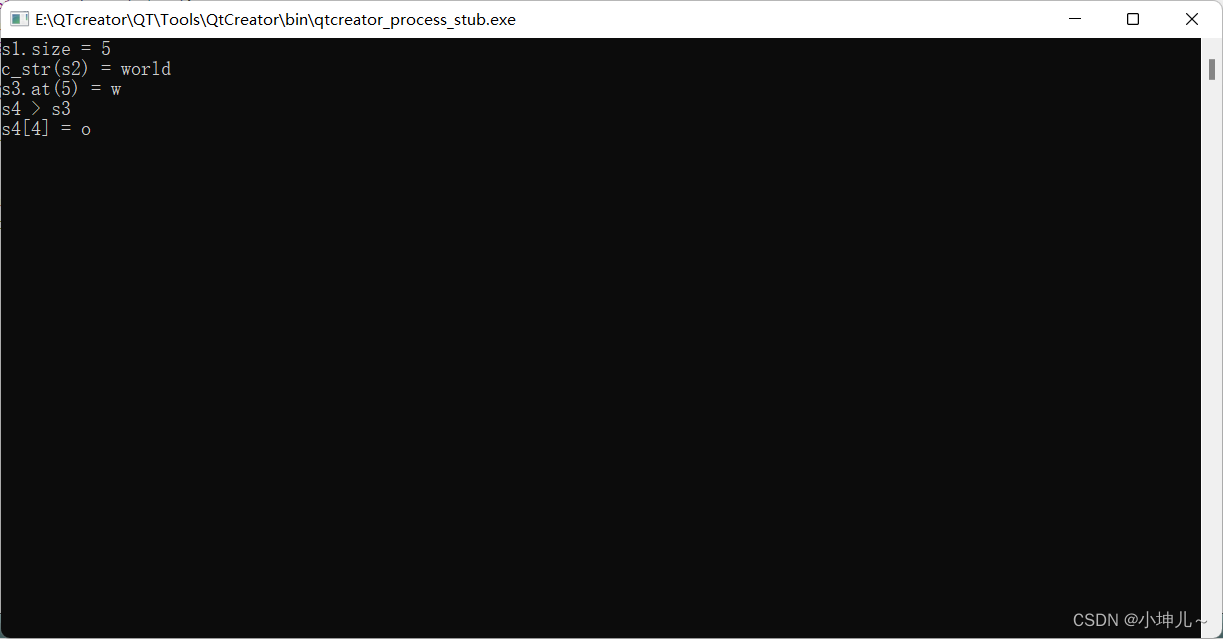# 作业

02mystring.h:

``````#ifndef __02MYSTRING_H__
#define __02MYSTRING_H__

#include <iostream>
#include <cstring>

using namespace std;

class myString{
private:
char *str;
int size;
public:
//无参构造
myString();
//有参构造
myString(const char *s);
//拷贝构造
myString(const myString &other);
//析构函数
~myString();
//拷贝赋值函数
myString & operator=(const myString &other);
//判空
bool empty()const;
//size
size_t string_size()const;
//c_str
const char *c_str()const;
//at
char &at(int pos);
//+运算符
const myString operator+(const myString &s)const;
//+=运算符
myString &operator+=(const myString &s);
//>运算符
bool operator>(const myString &s)const;
//[]运算符
char operator[](int index);
};

#endif // 02MYSTRING_H
``````

02mystring.cpp:

``````#include <02mystring.h>
//无参构造
myString::myString():size(10){
str = new char[size];
strcpy(str,"");
//cout<<"无参构造函数"<<endl;
}
//有参构造
myString::myString(const char *s){
size = strlen(s);
str = new char[size+1];
strcpy(str,s);
//cout<<"有参构造函数"<<endl;
}
//拷贝构造
myString::myString(const myString &other):size(other.size){
str = new char[other.size+1];
strcpy(str,other.str);
//cout<<"拷贝构造函数"<<endl;
}
//析构函数
myString::~myString(){
delete []str;
str = nullptr;
//cout<<"析构函数"<<endl;
}
//拷贝赋值函数
myString & myString::operator=(const myString &other){
size = other.size;
strcpy(str,other.str);
//cout<<"拷贝赋值函数"<<endl;
return *this;
}
//判空
bool myString::empty()const{
return size == 0;
}
//size
size_t myString::string_size()const{
return size;
}
//c_str
const char *myString::c_str()const{
return str;
}
//at
char &myString::at(int pos){
if(pos < 0 || pos >= size){
cout<<"pos出错啦！"<<endl;
return *str;             //不知道返回值些什么...嘻嘻，就返回第一个位置...
}
return *(str+pos);
}
//+运算符
const myString myString::operator+(const myString &s)const{
myString S;
delete []S.str;
S.size = size + s.size;
S.str = new char[S.size+1];
strcpy(S.str,str);
strcat(S.str,s.str);
return S;
}
//+=运算符
myString &myString::operator+=(const myString &s){
size += s.size;
strcat(str,s.str);
return *this;
}
//>运算符
bool myString::operator>(const myString &s)const{
return strcmp(str,s.str) > 0;
}
//[]运算符
char myString::operator[](int index){
return *(str+index);
}
``````

main.cpp:

``````#include <02mystring.h>

int main()
{
myString s1("hello");
myString s2("world");
myString s5;
cout<<"s1.size = "<<s1.string_size()<<endl;
cout<<"c_str(s2) = "<<s2.c_str()<<endl;
myString s3 = s1 + s2;
cout<<"s3.at(5) = "<<s3.at(5)<<endl;
myString s4("nihao");
s4 += s2;
if(s4 > s3){
cout<<"s4 > s3"<<endl;
}else {
cout<<"s4 <= s3"<<endl;
}
cout<<"s4 = "<<s4<<endl;
return 0;
}
``````

效果图:# 一、友元（friend）

### 1.1 友元函数

1> 普通的类的非成员函数，是无权访问类中的受保护成员以及私有成员的，但是，将一个函数设置成友元函数，那么该函数可以访问该类中的所有权限下的成员，

2> 友元函数分为全局函数作为友元函数和其他类的成员函数作为友元函数

3> 全局函数作为友元函数格式：在类内进行全局函数的声明：friend 函数头；

4> 其他类的成员函数作为友元函数，声明格式：friend 其他类：：函数名（形参列表）;

### 1.2 友元类

``````#include <iostream>

using namespace std;
class Stu;             //对类的前置声明

void fun(Stu &s);            //将函数进行前置声明

class Teacher
{
private:
string name;
string subject;          //科目

public:
Teacher() {}
Teacher(string n, string s):name(n), subject(s) {}

void display(Stu &s);             //类内声明，类外定义

};

class Stu
{
private:
string name;
int age;
double score;

public:
Stu() {}
Stu(string n, int a, double s):name(n),age(a),score(s) {}

//类中的成员函数，可以直接使用所有权限下的成员
void show()
{
cout<<"name = "<<name<<"  age = "<<age<<"  score = "<<score<<endl;
}

//将全局函数声明成友元函数,那么该全局函数可以访问该类中的所有权限下的成员
friend void fun(Stu &s);

//将老师类中的成员函数设置成友元函数
//friend void Teacher::display(Stu &s);

//将整个老师类都声明成友元类
friend class Teacher;
};

//定义一个全局函数
void fun(Stu &s)
{
cout<<"name = "<<s.name<<"  age = "<<s.age<<"  score = "<<s.score<<endl;
}

//将老师类中的成员函数类外定义
void Teacher::display(Stu &s)
{
cout<<"my_name = "<<name<<endl;
cout<<"my_subject = "<<subject<<endl;

cout<<"stu_name = "<<s.name<<endl;
}

int main()
{
Stu s("zhangpp", 18, 90);
fun(s);

Teacher t("zhansan", "C++");
t.display(s);

return 0;
}
``````

### 1.3 友元的总结

1. 友元是单向的，A将B设置成友元，则A允许B访问自己的成员，而B不一定允许A访问其成员
2. 友元不具有传递性：A是B的朋友，B是C的朋友，那么A不一定是C的朋友
3. 友元不具有继承性：父类的朋友不一定是子类的朋友
4. 友元破坏了类的封装性，使得访问权限形同虚设，所以不在万不得已的情况下，尽可能不使用友元
5. 必须使用友元的情况：插入和提取运算符重载时，需要使用全局函数作为友元函数

# 二、常成员（const）

### 2.1 常成员函数

1> 在定义成员函数后，加关键字const，那么该函数就是常成员函数

2> 在常成员函数中，不能修改成员变量的值，保护成员变量不被修改，但是可以使用成员变量

3> 常成员函数和同名的非常成员函数，即使参数种类和个数等同，也构成重载关系，原因是this指针的类型不同

非常成员函数中this: 类名 * const this;

常成员函数中this：const 类名 * const this;

4> 非常对象，调用成员函数时，优先调用非常成员函数，如果没有非常成员函数，那么就调用同名的常成员函数

### 2.2 常对象

1> 定义对象时，加关键字const: const 类名 对象名；

2> 常对象只能调用常成员函数，不能调用非常成员函数

### 2.3 mutable关键字

``````#include <iostream>

using namespace std;
class Stu
{
private:
string name;
mutable int age;            //由mutable修饰的成员变量，可以在常成员函数中被修改
double score;

public:
Stu() {}
Stu(string n, int a, double s):name(n),age(a),score(s) {}

//类中的成员函数，可以直接使用所有权限下的成员
void show()const            //this原型：const 类名  * const this;
{
//this->score = 100;              //更改成员变量的值
this->age = 16;            //由于该成员变量由mutable修饰，取消了其常属性
cout<<"name = "<<name<<"  age = "<<age<<"  score = "<<this->score<<endl;
}

//定义同名的非常成员函数
void show()                   //this原型：类名  * const this;
{
this->score = 100;              //更改成员变量的值
cout<<"name = "<<name<<"  age = "<<age<<"  score = "<<score<<endl;
}
};

int main()
{
Stu s1("zhangs", 18, 90);
//s1.show();                      //100

const Stu s2("lisi", 20, 50);        //该对象为常对象
//s2.show();

//定义常引用，目标为非常对象
const Stu &ref = s1;

ref.show();            // 常引用也有常属性，调用成员函数时，只能调用常成员函数，不能调用非常成员函数
s1.show();            //调用非常成员函数

return 0;
}``````

# 三、运算符重载（operator）

### 3.3 调用原则及调用时机

1> 调用时机：使用该运算符时，系统自动调用，无需手动调用

2> 调用原则：左调右参 （运算符的左侧是函数调用者，右侧是该函数的参数） 例如：a = b; //a.operator=(b)

### 3.5 算术类运算符重载

1> 种类：+、-、*、/、%

2> 表达式格式：L # R //L表示左操作数 #表示运算符 R表示右操作数

3> 左操作数：既可以是左值也可以是右值，运算过程中不会被修改

4> 右操作数：既可以是左值也可以是右值，运算过程中不会被修改

5> 结果：结果是一个同类的右值，不能被改变

6> 定义格式：

### 3.6 关系类运算符重载

1> 种类：>、=、

2> 表达式格式：L # R //L表示左操作数 #表示运算符 R表示右操作数

3> 左操作数：既可以是左值也可以是右值，运算过程中不会被修改

4> 右操作数：既可以是左值也可以是右值，运算过程中不会被修改

5> 结果：bool类型，表示真假

6> 定义格式：

### 3.7 赋值类运算符重载

1> 种类：=、+=、-=、*=、/=、%=

2> 表达式格式：L # R //L表示左操作数 #表示运算符 R表示右操作数

3> 左操作数：只能是左值

4> 右操作数：既可以是左值也可以是右值，运算过程中不会被修改

5> 结果：自身的引用

6> 定义格式：

### 3.8 单目运算符重载

1> 种类：！、~、*、&、-

2> 表达式格式： # O                 // #表示运算符 O表示操作数

3> 操作数：既可以是左值也可以是右值，运算过程中不能更改

4> 结果：同类的右值

5> 定义格式：

``````#include <iostream>

using namespace std;

//定义一个复数类 5 + 3i
class Complex
{
private:
int real;         //实部
int vir;          //虚部
public:
Complex() {}
Complex(int r, int v):real(r), vir(v) {}         //有参构造
//定义展示函数
void show()
{
if(vir>=0)
{
cout<<real<<" + "<<vir<<"i"<<endl;
}else
{
cout<<real<<vir<<"i"<<endl;
}
}

//全局函数版实现加运算符重载
friend const Complex operator+ (const Complex &L, const Complex &R);

//成员函数版实现运算符重载
const  Complex  operator- ( const Complex  &R)const
{
Complex c;
c.real = this->real - R.real;
c.vir = this->vir - R.vir;
return c;
}

//成员函数版实现关系运算符的重载：实部>实部  && 虚部>虚部
bool operator>(const Complex &R)const
{
return this->real>R.real&&this->vir>R.vir;
}

//重载中括号运算符
int & operator[](int index)
{
if(index == 0)
{
return real;          //返回实部
}else if(index == 1)
{
return vir;           //返回虚部
}
}

//重载+=运算符：实部+=实部   虚部+=虚部
Complex & operator+=(const Complex &R)
{
this->real += R.real;
this->vir += R.vir;
return *this;                //返回自身的引用
}

//重载负号运算符： 实部= -实部， 虚部 = -虚部
Complex operator-()
{
Complex c;
c.real = -this->real;
c.vir = -this->vir;
return c;
}
};

//全局函数版实现加号运算符重载：实部+实部  虚部+虚部
const Complex operator+ (const Complex &L, const Complex &R)
{
//定义一个临时空间
Complex c;

c.real = L.real + R.real;
c.vir = L.vir + R.vir;

return c;
}

int main()
{
Complex c1(5,3);
c1.show();                    //5+3i

Complex c2(2,-1);
c2.show();                      //2-1i

Complex c3 = c1-c2;             //调用加法运算符重载函数  c1.operator-(c2)

c3.show();                      //3+4i

if(c3 > c2)              //调用关系运算符重载函数
{
cout<<"yes"<<endl;
}else
{
cout<<"no"<<endl;
}

c3 = 5;               //将实部进行修改成5,调用中括号运算符重载
c3.show();                  //5+4i

c3 += c2;            //调用+=运算符重载函数
c3.show();             //7+3i

Complex c4 = -c3;      //调用-号运算符重载
c4.show();              //-7 - 3i
c3.show();            //7+3i

return 0;
}

``````

### 网站公告